# C program to perform addition, subtraction, multiplication, and division

In this article, we will learn about how to create a program that works on the famous four mathematical operations such as add, subtract, multiply, and divide in the following ways:

## Add, Subtract, Multiply, and Divide in C

The question is: Write a program in C that performs all four basic mathematical operations, such as addition, subtraction, multiplication, and division.

Here is the program in C that solves the above question, but it is only applicable for integer data.

```#include<stdio.h>
#include<conio.h>
int main()
{
int num1, num2, res;
printf("Enter any two number: ");
scanf("%d%d", &num1, &num2);
res = num1+num2;
res = num1-num2;
printf("\nSubtraction = %d", res);
res = num1*num2;
printf("\nMultiplication = %d", res);
res = num1/num2;
printf("\nDivision = %d", res);
getch();
return 0;
}```

The above program was written in the Code::Blocks IDE; therefore, after a successful compile and run, here is the initial output: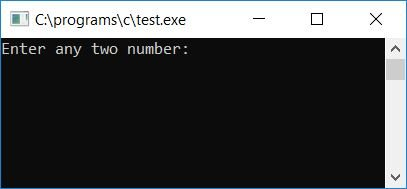Now supply any two numbers, say 90 and 45, as input and press the ENTER key to see the following output:#### Main steps used in the above program

Here are some of the main steps used in the above program:

• Initialize three variables of integer type, say num1, num2, and res.
• Variables num1 and num2 will be used to hold the value of the first and second numbers entered by the user at run-time. And the variable res will be used to store the value after performing the operation, such as add, subtract, multiply, and divide between two numbers.
• Receive the input from the user.
• Now initialize the addition result of the given two numbers stored in variables num1 and num2, respectively, to the variable res.
• Print the value of res as the sum of the two numbers given.
• Do the same for subtraction, multiplication, and division operations.

### Program to operate on real numbers

Now what if the user supplies any input number that is not a pure integer? Let's suppose that the user supplied any input, say 9.0 and 4.5, then the above program will not produce the correct result, as shown in the output given below: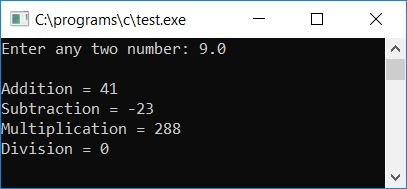To solve this problem, here is the modified version of the above program:

```#include<stdio.h>
#include<conio.h>
int main()
{
float num1, num2, res;
printf("Enter any two number: ");
scanf("%f%f", &num1, &num2);
res = num1+num2;
res = num1-num2;
printf("\nSubtraction = %.2f", res);
res = num1*num2;
printf("\nMultiplication = %.2f", res);
res = num1/num2;
printf("\nDivision = %.2f", res);
getch();
return 0;
}```

Here, we have changed the data type to "float" to handle real data or any real number that may include a decimal or not. After running the above program, enter any two numbers, say 9.0 and 4.5, and press the ENTER key to see the following output: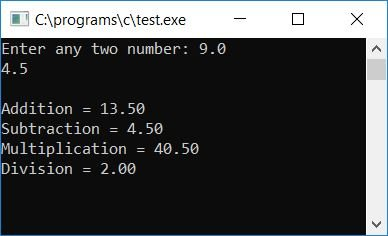To receive any floating-point (real number) data, use %f as a format specifier. To print the value of the res variable, we have placed. 2 between the format specifiers, say % and f, to print only two digits after the third decimal.

## Mathematical Operation Program in C using User-defined Function

Here is the program that works the same as the above program does, but internally, this program is programmed using user-defined functions. Let's take a look at it:

```#include<stdio.h>
#include<conio.h>
float Sub(float, float);
float Mul(float, float);
float Div(float, float);
int main()
{
float num1, num2, res;
printf("Enter any two number: ");
scanf("%f%f", &num1, &num2);
res = Sub(num1, num2);
printf("\nSubtraction = %.2f", res);
res = Mul(num1, num2);
printf("\nMultiplication = %.2f", res);
res = Div(num1, num2);
printf("\nDivision = %.2f", res);
getch();
return 0;
}
{
return a+b;
}
float Sub(float a, float b)
{
return a-b;
}
float Mul(float a, float b)
{
return a*b;
}
float Div(float a, float b)
{
return a/b;
}```

If you run the above program, it will work the same as the previous program in this article.

## Mathematical operation based on the user's choice

Now here is another version of almost the same program as previous programs, but this time, the program will not automatically operate and print the result of all four mathematical operations on the output screen.

Rather, this program will ask the user what to do, either to find addition or any other three mathematical operations such as subtraction, multiplication, and division. Let's take a look at it:

```#include<stdio.h>
#include<conio.h>
int main()
{
float num1, num2;
char ch;
printf("Enter First Number: ");
scanf("%f", &num1);
printf("Enter Second Number: ");
scanf("%f", &num2);
printf("Enter the Operator (+, -, *, /): ");
scanf(" %c", &ch);
if(ch=='+')
printf("Result = %0.2f", num1+num2);
else if(ch=='-')
printf("Result = %0.2f", num1-num2);
else if(ch=='*')
printf("Result = %0.2f", num1*num2);
else if(ch=='/')
printf("Result = %0.2f", num1/num2);
else
printf("Wrong Operator");
getch();
return 0;
}```

When the above C program is compiled and executed, it will produce the following result: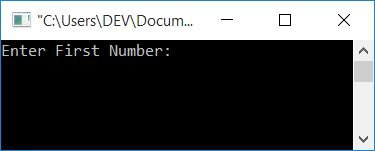Provide any input, say 45 as the first number and 14 as the second number, and press / as the division operator, then press the ENTER key to see the output as shown in the snapshot given below: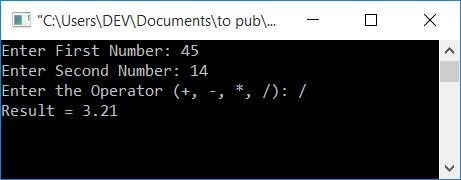#### The same program in different languages

C Quiz

« Previous Program Next Program »